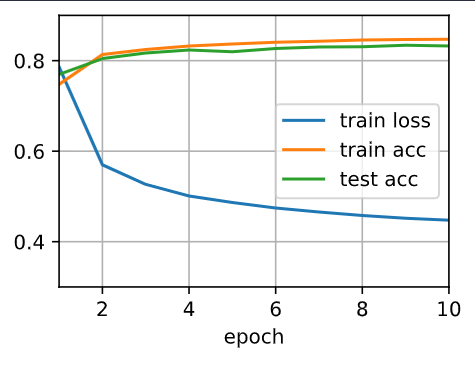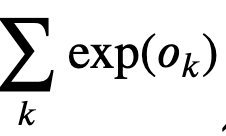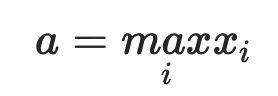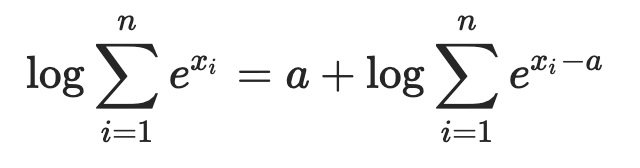# Concise Softmax Regression

1. batch_size num_epochs lr
2. It didn’t happen in my training. Why?I guess that the reason of “the test accuracy decrease again after a while” is that SGD updates too often.
Maybe we can try MBGD.

for more:

Hi @StevenJokes, we talked about the varied optimization algorithms in https://d2l.ai/chapter_optimization/index.html. Hopefully it helps!

I will learn it next time !

when testing the accuracy in concise Softmax regression, it seems that the max of the logits of the each example is used to determine the most probable class rather than the max of exp of logit. since that softmax is implemented in the cross entrophy loss, how did you do softmax in testing

Testing also has cross entrophy loss to calculate.@ccpvirus

where is it used? cant find it (reply should be at least 20 characters)

Hi @ccpvirus, please see my reply at Implementation of Multilayer Perceptron from Scratch.

Hi,
I have a question about the LogSumExp Trick. In the equation of " Softmax Implementation Revisited" section, we still have this partas part of LogSumExp trick. But this is still a exp() calculation of logit. Then how does this method solve of overflow problem? Thank you!

Great question @Philip_C! Since we are optimizing 𝑦̂ to a range of [0,1], we didn’t expect “exp()” to be a super large number. This LogSumExp trick is to deal the overflowed numerator and denominator.

1 Like

But I still have questions here. ∑𝑘exp(𝑜𝑘) is still calculating the exp() of the logit O, which can still be a large number, thus having the same overflow issue, right?

I want to give a more specific example about why I still don’t understand this trick. For example, let’s assume that we have a logit outcome coming from the model as `O = tensor([[-2180.4433, -1915.5579, 1683.3814, 633.9164, -1174.9501, -1144.8761, -1146.4674, -1423.6013, 606.3528, 1519.6882]])`, which will become the input of our new LogSumExp Trick loss function.
Now, in our loss function in the tutorial, we have the first part `Oj`, which do not require exp() calculation. Good. However, we still have the part `exp(Ok)` in the second part of the new loss function and this part still requires the exp() calculation of every element of the logit outcome `O`. Then we will still have the overflow issue, e.g. for the last element of `O`, we will have an overflow error for exp(1519.6882).

This is the part I am still confused. Thank you!

Hi @Philip_C, great question! Logsumexp is a trick for overflow. We usually pick the largest number in the tensor as `o_j`. i.e., we choosein. Please see more details here.

1 Like

Thank you! I got it now. I have to subtract the largest value a from it. I misunderstood it as I thought you don’t have to do that and it was another failed solution.

1 Like

Hi there,
I have copied the code precisely for both this tutorial and the previous one into an IDE (VS Code), and though it runs without errors, it does not produce any visualisations as depicted. It simply completes running silently. Are the graphs only available in a jupyter-like notebook environment?

### Exercises

1. Try adjusting the hyperparameters, such as the batch size, number of epochs, and learning
rate, to see what the results are.
• changing batch_Size increases time, more epochs trainingaccuracy is more or less constant, learning rate we have to try, changing to 1 loss increases, 0.01 converges slowly.
1. Increase the number of epochs for training. Why might the test accuracy decrease after a
while? How could we fix this?
• maybe overfitting